Do you like the article?
Share it with others —
Use new possibilities of MetaTrader 5

#### Similar articles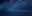# Fix PriceAction Stoploss or Fixed RSI (Smart StopLoss)

MetaTrader 5Examples | 25 November 2021, 13:0732 14418### Introduction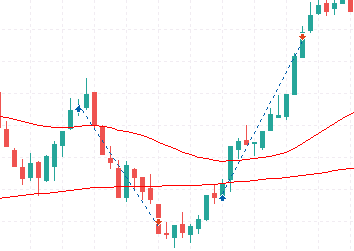Fig 1.1. Stop-loss Hunt

Stop-loss hunt is mostly associated with fixed or trailing stop-loss on the price action or candlestick chart. If there are no stop-loss on the price action chart, there will be no stop-loss hunt. But zero stop-loss is equal to the probability of blowing your trading account (which is equal to one (1)).

### RSI Stop-loss

The RSI oscillator is a replica of the price action line chart plotted on a limit window of 100 to 0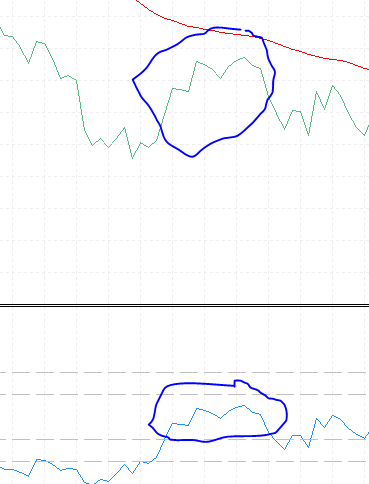Fig 1.2. RSI and Line Chart

If the RSI indicator and the Line Chart are very similar, then making use of the RSI indicator as a smart stop-loss might reduce the risk of stop-loss hunts.

### Aim:

My aim here is to verify if the use of RSI stop-loss would be able to reduce stop-loss hunt and above all be profitable in the long run.

### Objective:

Two same strategies will be compared: one with stop-loss set on the price action chart, and the other with stop-loss set on the RSI indicator.

### Classic Stop-Loss on price action chart

For the First EA with fixed stop-loss on the price action. Below are the requirements for the strategy

Parameters

Description

Used Indicator

MACD (12,26,9)

Used Indicator

Moving Average (200)

Used Indicator

Moving Average (50)

Used Indicator

ATR (5)

Time Frame

1 min

If the Moving Average (50) is above the Moving Average (200) and the MACD line is greater than Signal line when both the MACD line and the Signal line are below zero

Entry for Sell

If the Moving Average (50) is below the Moving Average (200) and the MACD line is less than Signal line when both the MACD line and the Signal line are above zero

Exit

Take profit and Stop-loss (1:2R).

The stop-loss for buy condition is the lowest of twenty (20) candles after entry minus the ATR (5) value

And the stop-loss for sell condition is the highest of twenty (20) candles after entry plus the ATR (5) value

Graphical representation is shown below.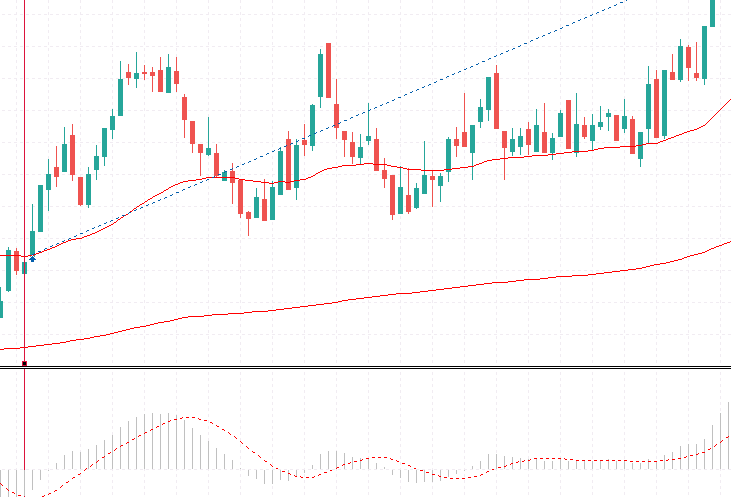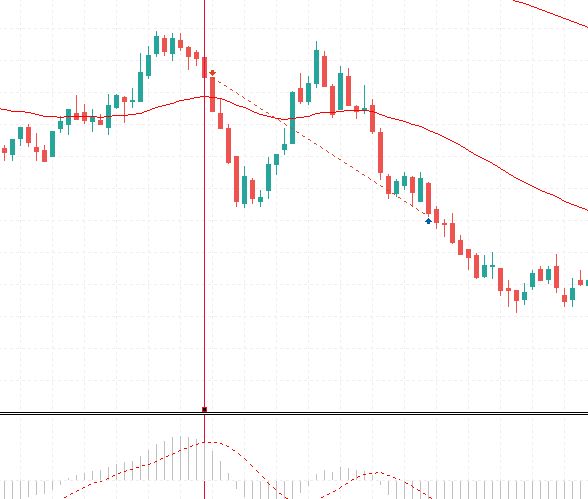Fig 2.2 Sell Entry

### Code

The first part of the code is mainly for variable declaration and input data. All the indicator handler variable were declared here.

### ```#property copyright "Copyright 2021, MetaQuotes Ltd." #property link      "https://www.mql5.com" #property version   "1.00" //+------------------------------------------------------------------+ //| Expert initialization function                                   | //+------------------------------------------------------------------+ #include<Trade\Trade.mqh> CTrade trade; int MATrend; int MADirection; int MACD; int ATR; input int afi;// ----------RiskAmount------------ input double risk = 0.02; //% Amount to risk input int atrValue = 20; // ATR VAlue input int ai;// ----------Moving Average inputs------------ input int movAvgTrend = 200;// Moving Average Trend input int movAvgDirection = 50;//moving Average for trend Direction; input int i;// -----------MACD inputs----------------------- input int fast = 12;// Macd Fast input int slow = 26; //Macd Slow input int signal = 9; //Signal Line```

Other variable declared

```double pipValue  = 0.0;//

double Balance; // For the Current Balance```

The variables were assigned to each handler in the init() function

```int OnInit()
{
//---
//Moving Averages Indicators''
MATrend = iMA(_Symbol,_Period,movAvgTrend,0,MODE_SMA,PRICE_CLOSE); //Moving Average 200
MADirection = iMA(_Symbol,_Period,movAvgDirection,0,MODE_EMA,PRICE_CLOSE); //Moving Average 50
//MACD
MACD = iMACD(_Symbol,_Period,fast,slow,signal,PRICE_CLOSE);//MACD
//ATR
ATR = iATR(_Symbol,_Period,atrValue);
//---
point=_Point;
double Digits=_Digits;

if((_Digits==3) || (_Digits==5))
{
point*=10;
}
return(INIT_SUCCEEDED);
}```

The code on how the strategy runs is shown below

```void Strategy() {
MqlRates priceAction[];
ArraySetAsSeries(priceAction,true);
int priceData = CopyRates(_Symbol,_Period,0,200,priceAction);

double maTrend[]; ArraySetAsSeries(maTrend,true);
CopyBuffer(MATrend,0,0,200,maTrend); //200 MA

double macd[]; ArraySetAsSeries(macd,true);
CopyBuffer(MACD,0,0,200,macd);  //MACD

double macds[]; ArraySetAsSeries(macds,true);
CopyBuffer(MACD,1,0,200,macds);  //MACD_Signal Line

double Bid  = NormalizeDouble(SymbolInfoDouble(_Symbol,SYMBOL_BID),_Digits);

bool macd_bZero = macds<0&&macd<0; //MacD Signal Line is less than Zero
bool macd_cross = macd>macds;// Macd Crosses the signal line

if (macd_bZero && macd_cross) {
}

//Sell; DownTrend

bool macd_bZero = macds>0&&macd>0;; //MacD Signal Line is less than Zero
bool macd_cross = macd<macds;// Macd Crosses the signal line

if (macd_bZero && macd_cross) {
}

}

return;
}

Sell(Bid);
}

}```

```void Buy(double Ask) {
double atr[]; ArraySetAsSeries(atr,true); //This array is use to store all ATR value to the last closed bar
CopyBuffer(ATR,0,0,200,atr); // This method copy the buffer value of the ATR indicator into the array (200 buffered data)

theLotsize =  NormalizeDouble((Balance*risk)/((MathAbs(Ask-((stoplossforBuy(20)-atr)))*100)*pipValue),2); // This Calculate the lotsize using the % to risk

}
void Sell(double Bid) {
double atr[]; ArraySetAsSeries(atr,true); //This array is use to store all ATR value to the last closed bar
CopyBuffer(ATR,0,0,200,atr); // This method copy the buffer value of the ATR indicator into the array (200 buffered data)
theLotsize =  NormalizeDouble((Balance*risk)/((MathAbs(Bid-((stoplossforSell(20)+atr)))*100)*pipValue),2); // This Calculate the lotsize using the % to risk
trade.Sell(theLotsize,_Symbol,Bid,(stoplossforSell(20)+atr),Bid-(2*MathAbs(((stoplossforSell(20)+atr))-Bid)),NULL); //Sell Entry with zero stoploss && take profit is twice the distance between the entry and the highest candle

}```

From the above codes we called two methods which are the stoplossforSell(int num) and the stoplossforBuy(int num). These two methods are meant specifically for identifying the highest and lowest candle of the assigned number respectively after the trade entry is triggered. E.g stoplossforSell(20) returns the highest candle among 20 previous candles before the entry.

```double stoplossforBuy(int numcandle) {
int LowestCandle;

//Create array for candle lows
double low[];

//Sort Candle from current downward
ArraySetAsSeries(low,true);

//Copy all lows for 100 candle
CopyLow(_Symbol,_Period,0,numcandle,low);

//Calculate the lowest candle
LowestCandle = ArrayMinimum(low,0,numcandle);

//Create array of price
MqlRates PriceInfo[];

ArraySetAsSeries(PriceInfo,true);

//Copy price data to array

int Data = CopyRates(Symbol(),Period(),0,Bars(Symbol(),Period()),PriceInfo);

return PriceInfo[LowestCandle].low;

}
double stoplossforSell(int numcandle) {
int HighestCandle;
double High[];

//Sort array downward from current candle
ArraySetAsSeries(High,true);

//Fill array with data for 100 candle
CopyHigh(_Symbol,_Period,0,numcandle,High);

//calculate highest candle
HighestCandle = ArrayMaximum(High,0,numcandle);

//Create array for price
MqlRates PriceInformation[];
ArraySetAsSeries(PriceInformation,true);

//Copy price data to array
int Data = CopyRates(Symbol(),Period(),0,Bars(Symbol(),Period()),PriceInformation);

return PriceInformation[HighestCandle].high;

}```

This EA enters trade one at a time by checking if there is an open trade or not. If there are no open trades, then the strategy method is called.

```void OnTick()
{
//---

Balance = AccountInfoDouble(ACCOUNT_BALANCE);

if (PositionsTotal()==0) {
Strategy();
}
}```

RSI Stop-Loss on the RSI indicator

For the Second EA with fixed stop-loss on the RSI Indicator. Below are the requirements for the strategy.

 Parameters Description Used Indicator MACD (12,26,9) Used Indicator Moving Average (200) Used Indicator Moving Average (50) Used Indicator ATR (5) Time Frame 1 min Entry for Buy If the Moving Average (50) is above the Moving Average (200) and the MACD line is greater than Signal line when both the MACD line and the Signal line are below zero Entry for Sell If the Moving Average (50) is below the Moving Average (200) and the MACD line is less than Signal line when both the MACD line and the Signal line are above zero Exit Take profit. The stop-loss for buy condition is the lowest of twenty (10) RSI Value after Entry And the stop-loss for sell condition is the highest of twenty (10) RSI Value after Entry   If the RSI crosses the highest or lowest RSI, the trade is closed.

Line is drawn on the RSI Stop loss for easy visualization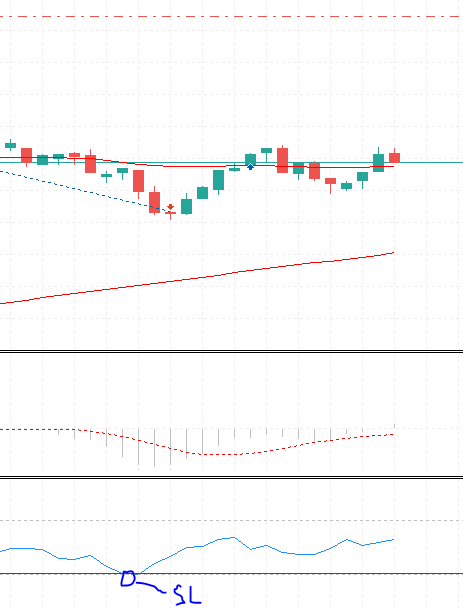The trade closes when the current RSI value is less than the Stop-loss line on the RSI window.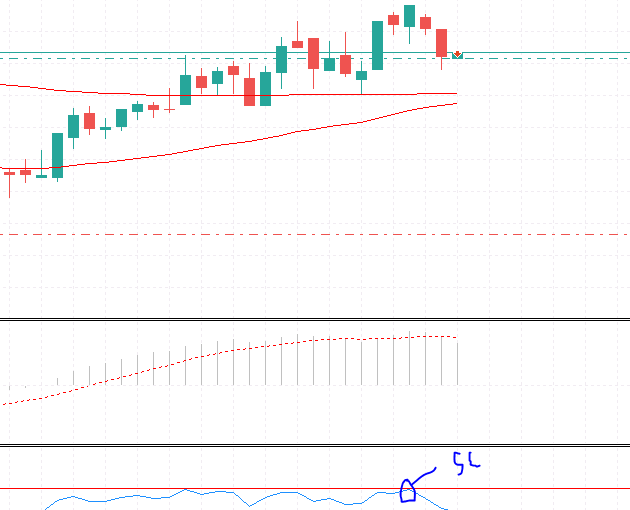Fig 2.4. Sell Trade with Stop-loss at the RSI

The trade closes when the current RSI value is greater than the Stop-loss line on the RSI window.

### Code

The first part of the code is mainly for variable declaration and input data. Similar to the first indicator with few additions shown below

```int RSI;
input int rsi = 5; // RSI VAlue
double lowestrsiValue = 100;
double highestrsiValue = 0.0;```

Similarly, the onint() method is also similar with few additions as shown below

```int OnInit()
{
//RSI
RSI = iRSI(_Symbol,_Period,rsi,PRICE_CLOSE);
}```

The code for the strategy is same as above. But the entry for both buy and sell has no stop loss in it because we will be using the RSI level as the stop-loss. Below are the code for the entries

```void Buy(double Ask) {
double atr[]; ArraySetAsSeries(atr,true); //This array is use to store all ATR value to the last closed bar
CopyBuffer(ATR,0,0,200,atr); // This method copy the buffer value of the ATR indicator into the array (200 buffered data)

theLotsize =  NormalizeDouble((Balance*risk)/((MathAbs(Ask-((stoplossforBuy(20)-atr)))*100)*pipValue),2); // This Calculate the lotsize using the % to risk

ObjectCreate(0,"sl",OBJ_HLINE,3,0,lowestRSI(10)); // Since our stoploss is zero we assign a smart stoploss on the rsi by drawing a line on the rsi window
Print("SL",lowestRSI(10));
}
void Sell(double Bid) {
double atr[]; ArraySetAsSeries(atr,true); //This array is use to store all ATR value to the last closed bar
CopyBuffer(ATR,0,0,200,atr); // This method copy the buffer value of the ATR indicator into the array (200 buffered data)

theLotsize =  NormalizeDouble((Balance*risk)/((MathAbs(Bid-((stoplossforSell(20)+atr)))*100)*pipValue),2);  // This Calculate the lotsize using the % to risk

ObjectCreate(0,"sl",OBJ_HLINE,3,0,highestRSI(10)); // Since our stoploss is zero we assign a smart stoploss on the rsi by drawing a line on the rsi window
trade.Sell(theLotsize,_Symbol,Bid,0,Bid-(2*MathAbs(((stoplossforSell(20)+atr))-Bid)),NULL);//Sell Entry with zero stoploss && take profit is twice the distance between the entry and the highest candle
Print("SL",highestRSI(10));
}```

The final part of the code that's different from the above EA is the methods that get the lowest and highest values of the RSI. This is called from the entries method above and uses the data to draw a line at the point of the lowest or highest RSI.

Note: The drawn line is deleted in the strategy method when there is no open position.

``` double lowestRSI(int count) {
double thersi[]; ArraySetAsSeries(thersi,true);
CopyBuffer(RSI,0,0,200,thersi);

for (int i = 0; i<count;i++) {

if (thersi[i]<lowestrsiValue) {
lowestrsiValue = thersi[i];
}
}
return lowestrsiValue;
}
//This method get the Highest RSI afer ENtry to set the smart Stoploss
double highestRSI(int count) {

double thersi[]; ArraySetAsSeries(thersi,true);
CopyBuffer(RSI,0,0,200,thersi);

for (int i = 0; i<count;i++) {

if (thersi[i]>highestrsiValue) {
highestrsiValue = thersi[i];
}
}
return highestrsiValue;
}```

####

Now that the EAs are set and complied, we can proceed to testing both EAs for the required result.

### Stop-Loss hunt test

In the section, the test and the result obtained from the simulation will be present. The first test to be carried out is to determine if the RSI STOP-LOSS EA is able to REDUCE the issue of stop-loss hunt while trading. It will be compared to the CLASSIC STOP-LOSS EA.

The tests are done on M1 timeframe.

### RSI STOP-LOSS EA ENTRY DATA

 Expert: MACD_Smart_Stoploss Symbol: Volatility 10 Index Period: M1 (2021.07.01 - 2021.07.15) Inputs: afi=0 risk=0.05 atrValue=20 rsi=14 ai=0 movAvgTrend=200 movAvgDirection=50 i=0 fast=12 slow=26 signal=9 Broker: Deriv Limited Currency: USD Initial Deposit: 500.00 Leverage: 1:500

### CLASSIC STOP-LOSS EA ENTRY DATA

 Expert: MACD_Cross_Stoploss Symbol: Volatility 10 Index Period: M1 (2021.07.01 - 2021.07.15) Inputs: afi=0 risk=0.05 atrValue=5 ai=0 movAvgTrend=200 movAvgDirection=50 i=0 fast=12 slow=26 signal=9risk reward=1:2 Broker: Deriv Limited Currency: USD Initial Deposit: 500.00 Leverage: 1:50

### RESULTS

Graphical representation of trades carried out by both EAs will be displayed below. A total of 3 trade samples were taken from each.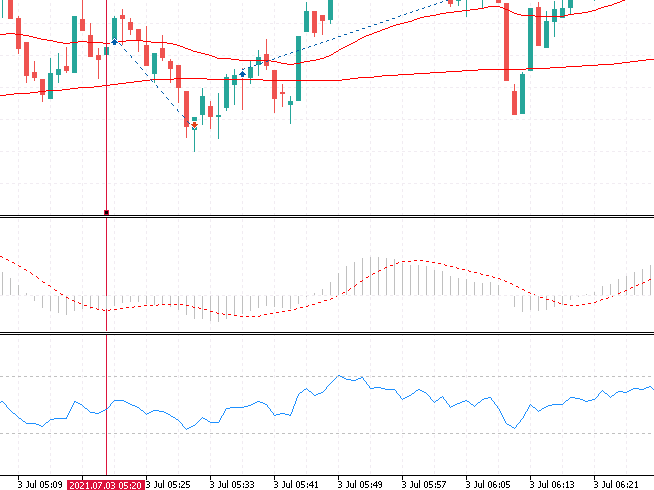Fig 3.1. Sample 1 from RSI STOP-LOSS EA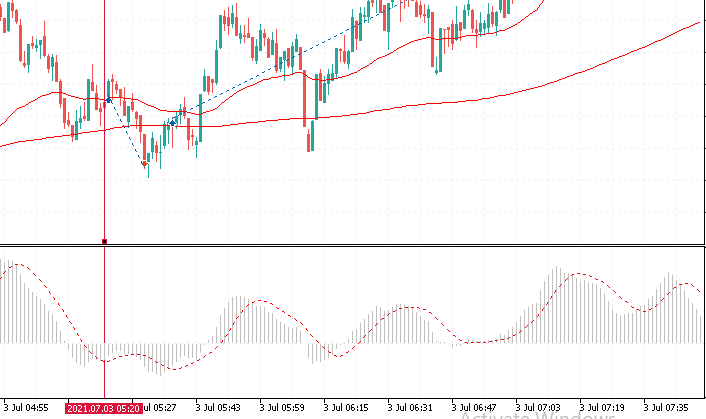Fig 3.1a. Sample 1 from CLASSIC STOP-LOSS EA

Fig 3.2. Sample 2 from RSI STOP-LOSS EA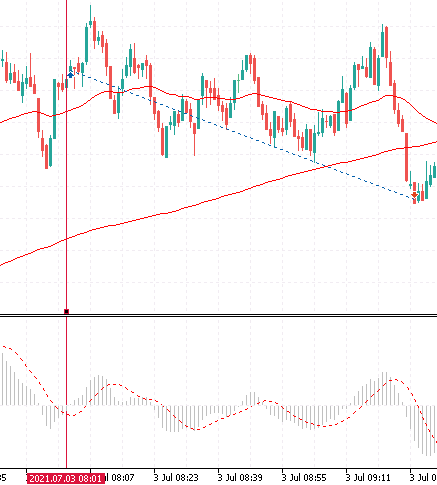Fig 3.2a. Sample 2 from CLASSIC STOP-LOSS EA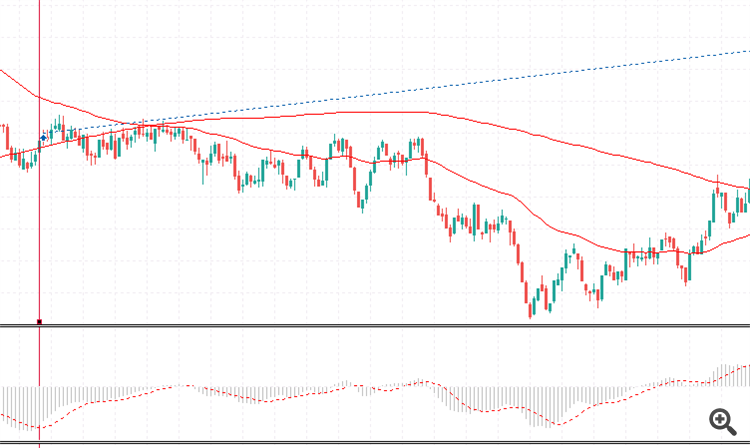Fig 3.3. Sample 3 from RSI STOP-LOSS EA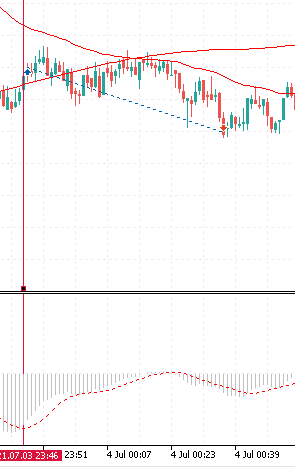Fig 3.3a. Sample 3 from CLASSIC STOP-LOSS EA

From the above comparison, it can be observed that the RSI STOP-LOSS EA did a great job at avoiding being hunted by the market compared to the classic Stop-Loss set on the price Action Chart.

### Profitability test

Now is it the time to ask the big question "Is this profitable?". Since the RSI STOP-LOSS EA did a great job at avoiding stop loss hunt, then it must be profitable and also have a higher win-rate because few stop-losses would be triggered compared to the classical stop-loss method. Logically this could be true. But to prove this theory a test must be conducted.

Similar data from the above test would be used for both tests. A back-test on both EA of above 100 trades would be carried out on M1 timeframe. Below are the results.

### RSI STOP-LOSS EA RESULTS

 Results History Quality: 100% Bars: 20160 Ticks: 603385 Symbols: 1 Total Net Profit: 327.71 Balance Drawdown Absolute: 288.96 Equity Drawdown Absolute: 367.85 Gross Profit: 3 525.74 Balance Drawdown Maximal: 483.90 (69.63%) Equity Drawdown Maximal: 523.24 (71.95%) Gross Loss: -3 198.03 Balance Drawdown Relative: 69.63% (483.90) Equity Drawdown Relative: 73.65% (369.45) Profit Factor: 1.10 Expected Payoff: 1.76 Margin Level: 317.21% Recovery Factor: 0.63 Sharpe Ratio: 0.08 Z-Score: 1.68 (90.70%) AHPR: 1.0070 (0.70%) LR Correlation: 0.51 OnTester result: 0 GHPR: 1.0027 (0.27%) LR Standard Error: 134.83 Total Trades: 186 Short Trades (won %): 94 (42.55%) Long Trades (won %): 92 (38.04%) Total Deals: 372 Profit Trades (% of total): 75 (40.32%) Loss Trades (% of total): 111 (59.68%) Largest profit trade: 85.26 Largest loss trade: -264.99 Average profit trade: 47.01 Average loss trade: -28.81 Maximum consecutive wins (\$): 5 (350.60) Maximum consecutive losses (\$): 6 (-255.81) Maximal consecutive profit (count): 350.60 (5) Maximal consecutive loss (count): -413.34 (5) Average consecutive wins: 2 Average consecutive losses: 2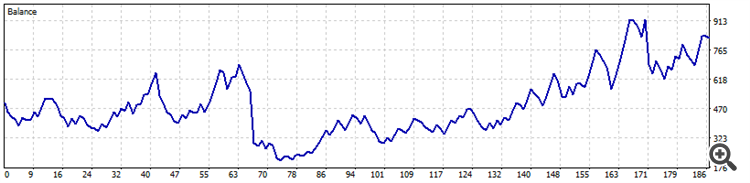Fig 3.4 Equity curve for RSI Stop-loss EA

### CLASSIC STOP-LOSS EA RESULTS

 Results History Quality: 100% Bars: 20160 Ticks: 603385 Symbols: 1 Total Net Profit: 3 672.06 Balance Drawdown Absolute: 215.45 Equity Drawdown Absolute: 217.30 Gross Profit: 10 635.21 Balance Drawdown Maximal: 829.54 (19.27%) Equity Drawdown Maximal: 1 159.20 (25.59%) Gross Loss: -6 963.15 Balance Drawdown Relative: 48.76% (270.82) Equity Drawdown Relative: 51.81% (303.90) Profit Factor: 1.53 Expected Payoff: 15.97 Margin Level: 274.21% Recovery Factor: 3.17 Sharpe Ratio: 0.16 Z-Score: -0.14 (11.13%) AHPR: 1.0120 (1.20%) LR Correlation: 0.80 OnTester result: 0 GHPR: 1.0093 (0.93%) LR Standard Error: 545.00 Total Trades: 230 Short Trades (won %): 107 (44.86%) Long Trades (won %): 123 (38.21%) Total Deals: 460 Profit Trades (% of total): 95 (41.30%) Loss Trades (% of total): 135 (58.70%) Largest profit trade: 392.11 Largest loss trade: -219.95 Average profit trade: 111.95 Average loss trade: -51.58 Maximum consecutive wins (\$): 6 (1 134.53) Maximum consecutive losses (\$): 9 (-211.43) Maximal consecutive profit (count): 1 134.53 (6) Maximal consecutive loss (count): -809.21 (4) Average consecutive wins: 2 Average consecutive losses: 2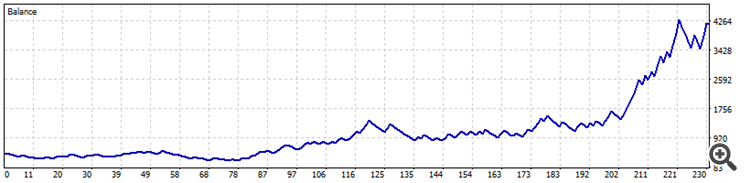Fig 3.5. Equity curve for Classic Stop loss EA

### Observation

Although both EAs were profitable at the end of the trading period, it was observed that the first EA (RSI Stop-Loss EA) had fewer losses in which some are huge losses.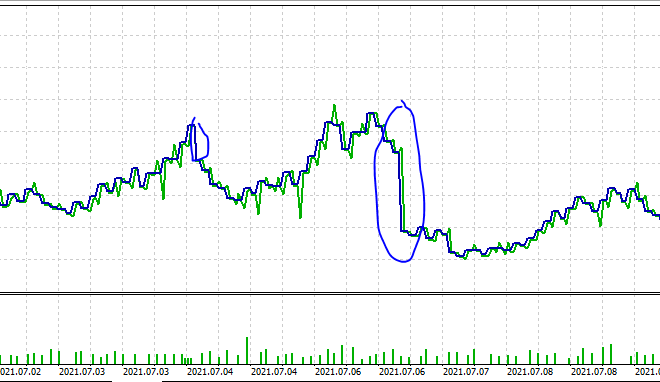Fig 3.6. Equity curve Loses

These losses might affect the overall profitability of the EA and also proper money management. On the other hand, the classic Stop loss EA had more losses and also made the most money at the end of the trading period.

### Conclusion and recommendation

Money Management is indeed the holy grail of trading. From the above experiment, the EA in which money management was fully implemented made the most profit with more loss. This is because of trade consistency. However, defining stop loss at the RSI does not fully give the same consistency for the first EA (RSI STOP-LOSS) as the risk amount varies.

### Recommendation

Hedging is one way of reducing the losses in the first (RSI STOP-LOSS) EA. This might give a more consistent risk amount and improve the profit in the long run.

Attached files |
Last comments | Go to discussion (18)| 12 Jan 2022 at 20:40
Eleni Anna Branou #:

Contact the author please, I wouldn't know.

i think the error is at _point or pipvalue in code| 12 Jan 2022 at 20:42
vwegba #:
The position is calculated from the price action, where you intends adding the stoploss. Since rsi is similar to line price action chart, we used the rsi instead of the price action chart. Also you are right that's the major issue with the strategy
hello dude.did you see my comments?whats the problem?| 11 Feb 2022 at 14:46
Essi afsoungar #:
hello dude.did you see my comments?whats the problem?

The bot was built specifically for synthetic pair. like the one used above was volatility 10 pair on meta trader 5.

For it to work on other pair few modifications are needed| 11 Feb 2022 at 17:32
Essi afsoungar #:
hello dude.did you see my comments?whats the problem?

To be able to use the bot on other pairs like the EURUSD pair you would need to multiply by 10,000 because 1 pip of the pair is 0.0001

below works for all pairs with pip value of 0.0001

for pip value of 0.01 thats JPY pairs you multiply by 100.

note the code is for mt5 only

Thank you| 24 Feb 2022 at 03:03
vwegba #:

To be able to use the bot on other pairs like the EURUSD pair you would need to multiply by 10,000 because 1 pip of the pair is 0.0001

below works for all pairs with pip value of 0.0001

for pip value of 0.01 thats JPY pairs you multiply by 100.

note the code is for mt5 only

Thank you

i think it has another problem... the expert sets tp and sl very too far from price.maybe needs another changes in code to work with euro usd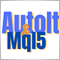Using AutoIt With MQL5
Short description. In this article we will explore scripting the MetraTrader 5 terminal by integrating MQL5 with AutoIt. In it we will cover how to automate various tasks by manipulating the terminals' user interface and also present a class that uses the AutoItX library.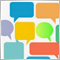Use MQL5.community channels and group chats
The MQL5.com website brings together traders from all over the world. Users publish articles, share free codes, sell products in the Market, perform Freelance orders and copy trading signals. You can communicate with them on the Forum, in trader chats and in MetaTrader channels.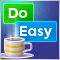Graphics in DoEasy library (Part 88): Graphical object collection — two-dimensional dynamic array for storing dynamically changing object properties
In this article, I will create a dynamic multidimensional array class with the ability to change the amount of data in any dimension. Based on the created class, I will create a two-dimensional dynamic array to store some dynamically changed properties of graphical objects.Graphics in DoEasy library (Part 87): Graphical object collection - managing object property modification on all open charts
In this article, I will continue my work on tracking standard graphical object events and create the functionality allowing users to control changes in the properties of graphical objects placed on any charts opened in the terminal.